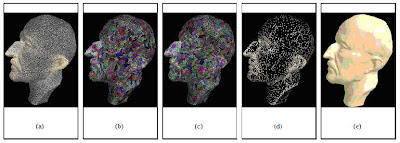## 30 September 2012

### Algorithm Developed For Resampling Framework Of Point Set Surfaces With Gaussian SpheresValid Gaussian surfaces are illustrated on the left while invalid ones on the right. Gaussian surfaces must enclose a 3-dimensional volume and have no boundaries. The figures on the right do not fully enclose a 3D volume, and have boundaries (red).
Gauss's Law states that the electric field on the edges of the surface of any closed 3 dimensional object is equal to the electrical charge inside it. For symmetrical objects such as spheres, applying Gauss's law is fairly straightforward.

To further understand what qualifies as a Gaussian surface, it is best to note that this kind of surface has no borders. A donut can qualify as a Gaussian surface since there are no borders or edges to it and the area inside the donut is enclosed. But a square surface or a hemispherical surface is not Gaussian because of the edges it has or that it does not enclose an area.

To apply Gauss's law to arbitrary shaped Gaussian surfaces, one can apply regular triangulation and the distribution of the surface. Using a point cloud, Gauss's law can assist in mathematically outlining the surface of the 3D objects through the X,Y, and Z vertices.

To do this, the surface element, called surfels, are used to reconstruct the whole surface of the object. Each surfel can be represented as one dot or disc in the surface area. By combining all this into densely packed surfels, the object can be imaged in a computer or mathematical environment. These surfel data make up the point cloud

Applications that utilize this technology in computer graphic systems such as medical imaging systems, nuclear or atomic rendering of particle systems, and the rendering surfaces of volumetric data.

Feature sensitive re-sampling of point set surfaces with Gaussian spheres

Feature sensitive re-sampling of point set surfaces is an important and challenging task in many computer graphics and geometric modeling applications. Professor MIAO Yongwei and his group at the College of Computer Science and Technology, Zhejiang University of Technology, set out to tackle this problem. Based on regular sampling of a Gaussian sphere and the mapping of surface normals onto the Gaussian sphere, they have presented an adaptive re-sampling framework for point set surfaces. The proposed re-sampling scheme can generate non-uniformly distributed discrete sample points for the underlying point sets in a feature sensitive manner. Their work, entitled "Feature sensitive re-sampling of point set surfaces with Gaussian spheres", has been published in SCIENCE CHINA Information Sciences, Vol. 55, 2012.

Video: Introduction to Gaussian Surfaces and Gauss's Law

Feature sensitive re-sampling of point set surfaces is an important and challenging task in many computer graphics and geometric modeling applications. Using regular triangulation of a Gaussian sphere and distribution of the surface normals over the Gaussian sphere, a Gaussian sphere based sampling scheme can be used to simplify the underlying point set surfaces in order to develop a simplified algorithm. The authors have proposed a novel framework of re-sampling point set surfaces, which can efficiently generate feature sensitive non-uniformly distributed discrete sample points.

The proposed algorithm takes as input a set of unstructured surfels rather than a discrete point cloud. A high-level outline of the proposed feature sensitive re-sampling algorithm based on a Gaussian sphere is summarized below.

1) Neighbor selection: Adaptive neighborhoods for each sample point are determined according to the normal deviation.This is a process flow of the feature sensitive re-sampling algorithm: (a) original uniform sampling of Max Planck model; (b) clusters after the naive index propagation step; (c) clusters after the optimized normalized rectification step; (d) feature sensitive sampling result of Max Planck model; (e) splat rendering result of simplified Max Planck model. Credit: ©Science China Press
2) Naive sampling: All sample points of the underlying model are clustered into regions using the proposed index propagation scheme, and then singleton points are merged into their nearest clusters;

3) Optimized sampling: The following two steps are iterated until convergence:
• cluster normalization: non-normalized clusters are split to generate normalized disk-like clusters
• singleton rejoining: singleton points located in the corner of Gaussian triangles are grouped with their nearest clusters.
4) Simplified surfel generation: Each cluster region is replaced by a representative surfel.

5) Splat rendering: The generated simplified models are finally rendered by elliptic splats.

The process flow of this feature sensitive re-sampling algorithm is outlined in the illustrated figure above.